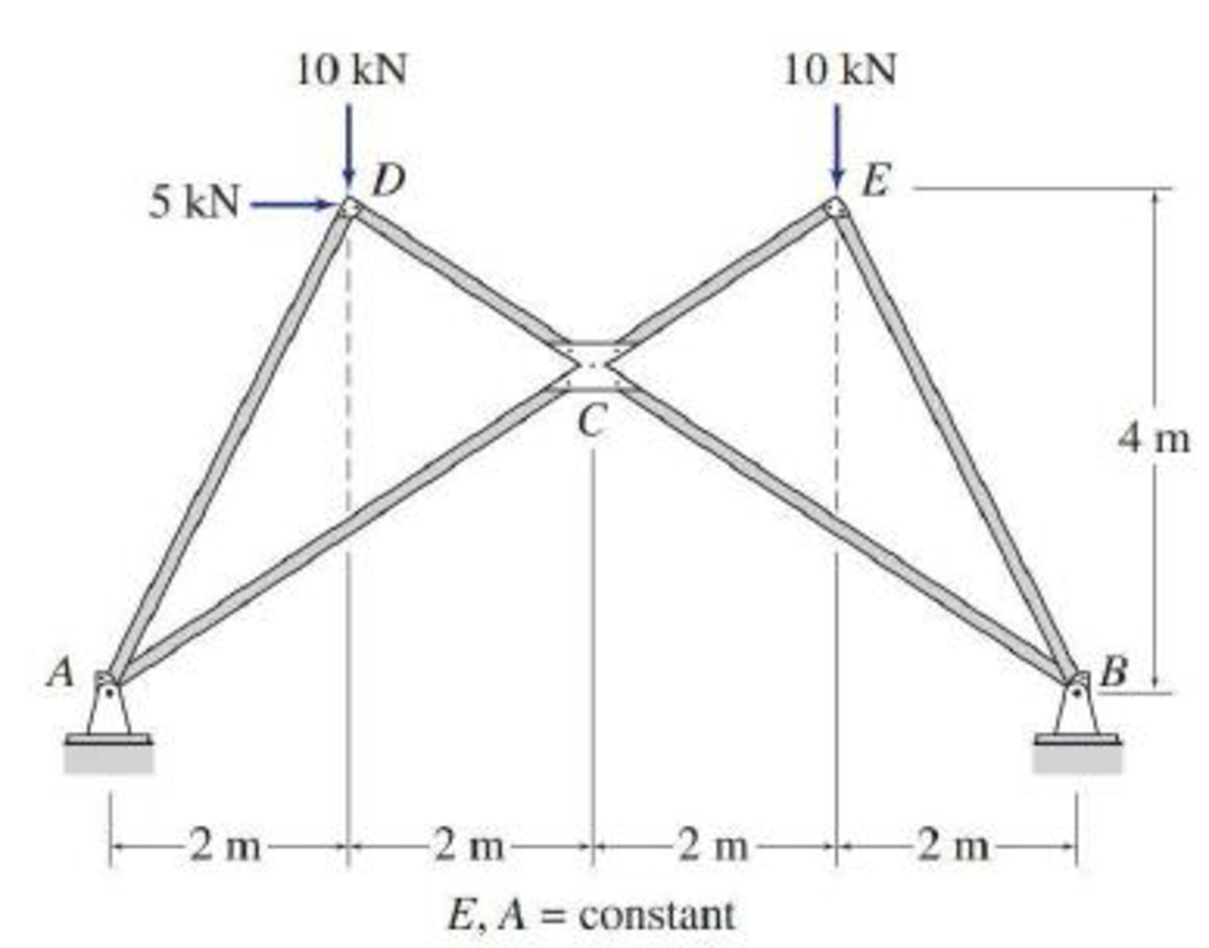# Determine the force in each member of the trusses shown in Figs. P10.1–P10.5 by utilizing structural symmetry. FIG. P10.5, P10.20

#### Solutions

Chapter
Section
Chapter 10, Problem 20P
Textbook Problem
47 views

## Determine the force in each member of the trusses shown in Figs. P10.1–P10.5 by utilizing structural symmetry.FIG. P10.5, P10.20

To determine

Find the force in each member of the truss using structural symmetry.

### Explanation of Solution

Given information:

The structure is given in the Figure.

The young’s modulus E and area A is constant.

Apply the sign conventions for calculating reactions, forces, and moments using the three equations of equilibrium as shown below.

• For summation of forces along x-direction is equal to zero (Fx=0), consider the forces acting towards right side as positive (+) and the forces acting towards left side as negative ().
• For summation of forces along y-direction is equal to zero (Fy=0), consider the upward force as positive (+) and the downward force as negative ().
• For summation of moment about a point is equal to zero (Matapoint=0), consider the clockwise moment as negative and the counter clockwise moment as positive.

Method of joints:

The negative value of force in any member indicates compression (C) and the positive value of force in any member indicates tension (T).

Calculation:

Refer the given structure.

The structure is symmetric with respect to the s axis.

Sketch the half loading for the given structure as shown in Figure 1.

Sketch the substructure with symmetric boundary conditions as shown in Figure 5.

Find the reactions and member end forces of substructure using equilibrium equations and to the left of s axis.

The member end forces to the right of s axis are obtained by the reflection.

Refer the given Figure.

The angle of AD to the horizontal is,

The angle of AE to the horizontal is,

tanAE=46AE=33.69°

Summation of forces along y-direction is equal to 0.

+Fy=010+Ay=0Ay=10kN

Summation of moments about A is equal to 0.

MA=0Cx×(4tan33.69°)2.5×410×2=02.667Cx=30Cx=11.25kN

Summation of forces along x-direction is equal to 0.

+Fx=02.5+AxCx=02.5+Ax11.25=0Ax=8.75kN

Consider joint A, find the force in the member AC and AD:

Summation of forces along y-direction is equal to 0.

Summation of forces along x-direction is equal to 0.

### Still sussing out bartleby?

Check out a sample textbook solution.

See a sample solution

#### The Solution to Your Study Problems

Bartleby provides explanations to thousands of textbook problems written by our experts, many with advanced degrees!

Get Started

Find more solutions based on key concepts
Give an example of a linear model in engineering.

Engineering Fundamentals: An Introduction to Engineering (MindTap Course List)

Differentiate among user names, passwords, passphrases, and pass codes.

Enhanced Discovering Computers 2017 (Shelly Cashman Series) (MindTap Course List)

What is an embedded system? Give three examples of such a system.

Principles of Information Systems (MindTap Course List)

What is privacy in an information security context?

Principles of Information Security (MindTap Course List)

What is calibration and why is it important?

Precision Machining Technology (MindTap Course List)

What are the disadvantages of the DDBMS?

Database Systems: Design, Implementation, & Management

What e the main principles of source document design?

Systems Analysis and Design (Shelly Cashman Series) (MindTap Course List)

If your motherboard supports ECC DDR3 memory, can you substitute non-ECC DDR3 memory?

A+ Guide to Hardware (Standalone Book) (MindTap Course List)

What changes can be made to successfully make a weld in a poorly fitted joint?

Welding: Principles and Applications (MindTap Course List)